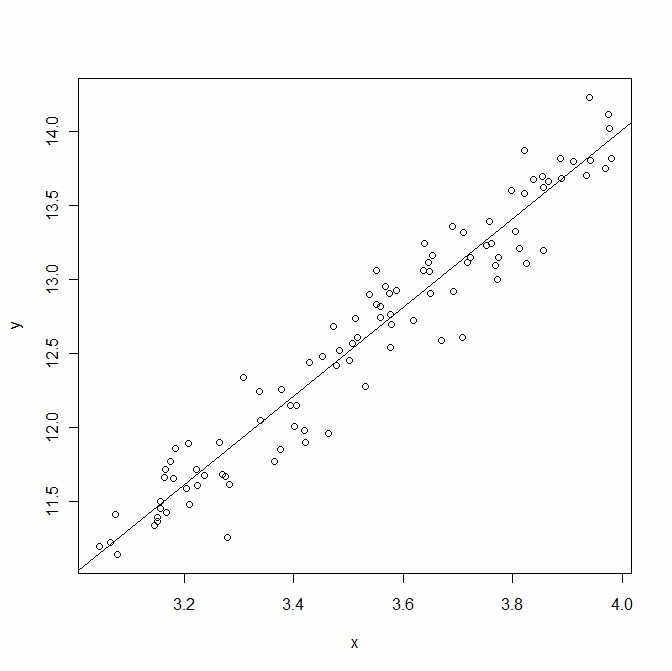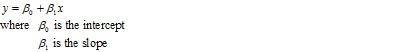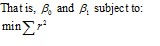# Regression

Statistical Analysis Techniques

Regression involves estimating the mathematical relationship between one variable called the response variable (or dependent variable), and one or more explanatory variables (or independent variables).

The explanatory variables are used to explain the response variable.  The response variable responds to changes in the explanatory variables.

Regression involves fitting a mathematical model to data.

The simplest example of a regression model is a straight line that passes through a set of points on a scatter plot.The x and y variables appear to have a linear relationship.  To summarise this relationship a regression model with the structure of a straight line (intercept and slope) is fitted to the data.The equation of the straight line was found by finding the coefficients values that minimise the sum of squared residuals (this method is known as ordinary least squares (OLS)).A residual is the difference between the observed response value (from the data) and estimated response value (found by plugging the observed explanatory value(s) into the model).After a regression model is fitted, it should then be diagnosed for faults; and if necessary, the model would be scrapped in favour of a better one.

Regression modelling has two purposes:
1.  To predict the response given a set of input values.
2.  To estimate the effect an explanatory variable has on the response variable.